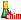Search:

# Ions and acids

## The simple ions

Taking or losing electrons, atoms tend often to adopt the structures $1s^2$ (first period) or $ns^2np^6$ (other periods) (octet rule). That leads us to the following elementary ions: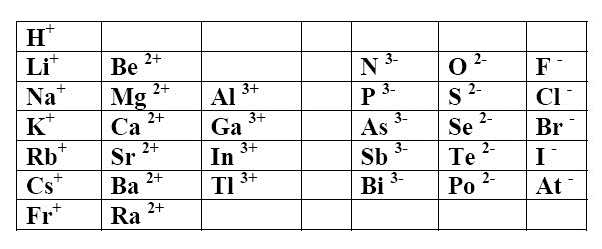Positive simple ions (cations) adopt the name of the element

Examples $Li^+$ is the lithium ion (or cation) $Ca^{2+}$ is the calcium ion (or cation)

Negative simple ions (anions) take the suffix -ide Exceptions: $O^{2-}$ is the oxide ion $P^{3-}$ is the phosphide ion $N^{3-}$ is the nitride ion $S^{2-}$ is the sulfide ion

Examples $Cl^-$ is the chloride ion (or anion) $Se^{2-}$ is the selenide ion (or anion)

All the preceding ions are colourless.

Ions of transition elements don't follow the octet rule. Sometimes they have a caracteristic color (which may be influenced by their surrounding):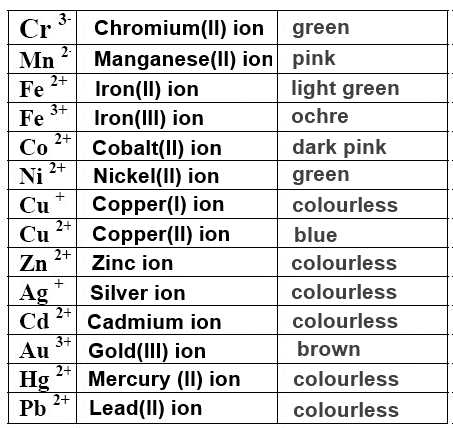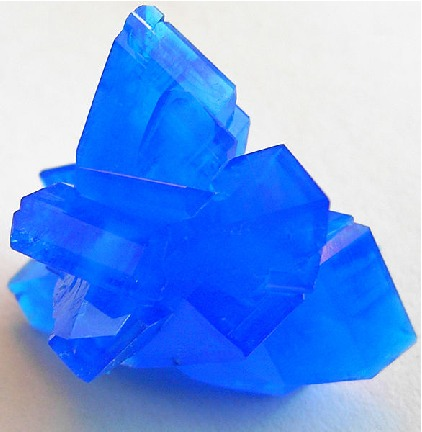Cristal containing the $Cu^{2+}$ ion

## Multiatomic ions

Multiatomic ions have sometimes a caracteristic color (which may be influenced by their surrounding: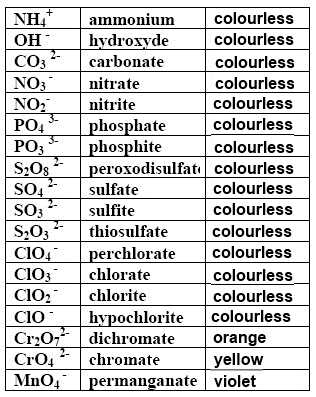Cristals containing the $Cr_2O_7^{2-}$ ion

## Acid ions

The addition of $H^+$ to an anion with multiple negative charge leads to an anion whose negative charge is diminished by one unit. Examples $HPO_4^{2-}$ is the (mono)hydrogen phosphate ion $H_2PO_4^{-}$ is the dihydrogen phosphate ion $HS^{-}$ is the hydrogen sulfide ion

Acid ions: Préfix: hydrogen Multiplicative factors : (mono), di tri (The prefix mono is optional)

## The acids

The addition of $H^+$ to an anion with a simple negative charge leads no more to an ion, but to neutral molecule of a substance called acid $HClO_4$ is the perchloric acid $HClO_3$ is the chloric acid $HClO_2$ is the chlorous acid $HClO$ is the hypochlorous acid $HCl$ is the chlorhydric acid

Anion with suffix -ide → acid with suffix -hydric Anion with suffix -ite → acid with suffix -ous Anion with suffix -ate → acid with suffix -ic

When acid molecules are introduced into water, they dissociate wholly or partially to form ions: Examples: $HCl$$H^+$ $+$ $Cl^-$ (complete dissociation: "strong" acid) $H_2CO_3$$H^+$ $+$ $HCO_3^-$ (partial dissociation: "weak " acid) $HF$$H^+$ $+$ $F^-$ (partial dissociation: "weak " acid)